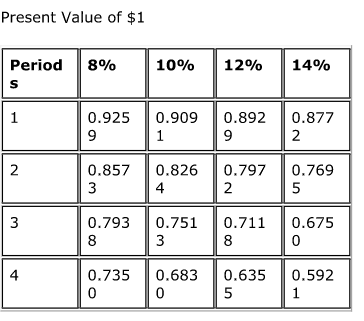# Problem: Compute the present value of $5,000 to be received one year from today if the interest rate is 10%, compounded annually. A)$4,545.50 B) $5,050.00 C)$4,500.00 D) $5,500.00 ###### FREE Expert Solution ###### Problem Details Compute the present value of$5,000 to be received one year from today if the interest rate is 10%, compounded annually.

A) $4,545.50 B)$5,050.00

C) $4,500.00 D)$5,500.00Frequently Asked Questions

What scientific concept do you need to know in order to solve this problem?

Our tutors have indicated that to solve this problem you will need to apply the Using Time Value of Money Tables concept. You can view video lessons to learn Using Time Value of Money Tables. Or if you need more Using Time Value of Money Tables practice, you can also practice Using Time Value of Money Tables practice problems.

What is the difficulty of this problem?

Our tutors rated the difficulty ofCompute the present value of \$5,000 to be received one year ...as low difficulty.